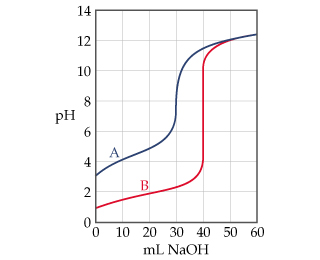# Problem: The graph below shows the titration curves for two monoprotic acids.Estimate the pKa of the weak acid.

###### FREE Expert Solution

We are asked to estimate the pKa of the weak acid from the titration curve.

A titration curve is a graphical representation of the pH of a solution during a titration

The figure below shows the titration of two monoprotic acids A and B.The equivalence point is the point in the titration curve where the amount of titrant added is enough to completely neutralize the analyte solution.

The half-equivalence point is the point at which the pH of the solution is equal to the dissociation constant (pKa) of the acid. This point is halfway between the equivalence point and the origin.

99% (254 ratings)###### Problem Details

The graph below shows the titration curves for two monoprotic acids.Estimate the pKa of the weak acid.